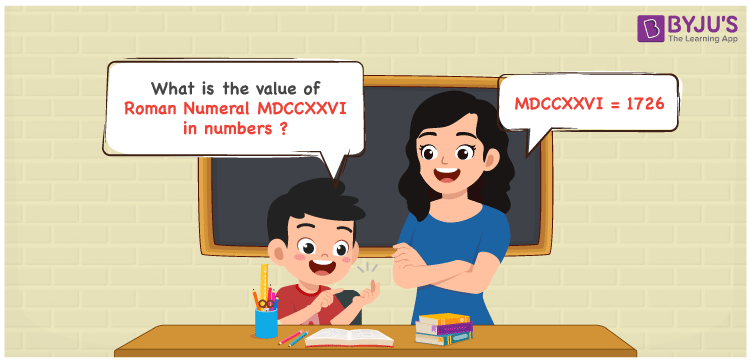Checkout JEE MAINS 2022 Question Paper Analysis : Checkout JEE MAINS 2022 Question Paper Analysis :

# MDCCXXVI Roman Numerals

MDCCXXVI Roman Numerals is 1726. The conversion steps to indicate the Roman numerals in numbers are shown in this article in a systematic way. The roman numerals topic is one of the important concepts which should be focused by the students from the beginning of the academic year. Therefore, the roman numerals MDCCXXVI is written as 1726 in numbers.

 Number Roman Numeral 1726 MDCCXXVI## How to Write MDCCXXVI Roman Numerals in Numbers?

This section has the steps of expansion used to write MDCCXXVI as 1726 in numbers.

MDCCXXVI = M + D + C + C + X + X + V + I

MDCCXXVI = 1000 + 500 + 100 + 100 + 10 + 10 + 5 + 1

MDCCXXVI = 1726

## Video Lesson on Roman Numerals## Frequently Asked Questions on MDCCXXVI Roman Numerals

### Why is MDCCXXVI the roman numerals of 1726?

We know that

M is the roman numerals of 1000

DCC is the roman numerals of 700

XX is the roman numerals of 20

VI is the roman numerals of 6

So MDCCXXVI is the roman numeral of 1726.

### Determine 1800 – 74.

We know that

1800 – 74 = 1726

Hence, 1800 – 74 is 1726 which is written as MDCCXXVI.

### Find the remainder if MDCCXXVI is divided by V.

We know that

MDCCXXVI = 1726

V = 5

The remainder if MDCCXXVI is divided by V is I.